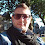## Friday, June 4, 2021

### The Central Limit Theorem, a hands-on introduction

The central limit theorem can be informally summarized in few words: The sum of x1, x2, ... xn samples from the same distribution is normally distributed, provided that n is big enough and that the distribution has a finite variance. to show this in an experimental way, let's define a function that sums n samples from the same distrubution for 100000 times:
```import numpy as np
import scipy.stats as sps
import matplotlib.pyplot as plt

def sum_random_variables(*kwarg, sp_distribution, n):
# returns the sum of n random samples
# drawn from sp_distribution
v = [sp_distribution.rvs(*kwarg, size=100000) for _ in range(n)]
return np.sum(v, axis=0)
```
This function takes in input the parameters of the distrubution, the function that implements the distrubution and n. It returns an array of 100000 elements, where each element is the sum of n samples. Given the Central Limit Theorem, we expect that the values in output are normally distributed if n is big enough. To verify this, let's consider a beta distribution with parameters alpha=1 and beta=2, run our function increasing n and plot the histogram of the values in output:
```plt.figure(figsize=(9, 3))
N = 5
for n in range(1, N):
plt.subplot(1, N-1, n)
s = sum_random_variables(1, 2, sp_distribution=sps.beta, n=n)
plt.hist(s, density=True)
plt.tight_layout()
```
On the far left we have the histogram with n=1 , the one with n=2 right next to it, and so on until n=4. With n=1 we have the original distribution, which is heavily skewed. With n=2 we have a distribution which is less skewed. When we reach n=4 we see that the distribution is almost symmetrical, resembling a normal distribution.

Let's do the same experiment using a uniform distribution:
```plt.figure(figsize=(9, 3))
for n in range(1, N):
plt.subplot(1, N-1, n)
s = sum_random_variables(1, 1, sp_distribution=sps.beta, n=n)
plt.hist(s, density=True)
plt.tight_layout()
```
Here we have that for n=2 the distribution is already symmetrical, resembling a triangle, and increasing n further we get closer to the shape of a Gaussian.

The same behaviour can be shown for discrete distributions. Here's what happens if we use the Bernoulli distribution:
```plt.figure(figsize=(9, 3))
for n in range(1, N):
plt.subplot(1, N-1, n)
s = sum_random_variables(.5, sp_distribution=sps.bernoulli, n=n)
plt.hist(s, bins=n+1, density=True, rwidth=.7)
plt.tight_layout()
```
We see again that for n=2 the distribution starts to be symmetrical and that the shape of a Gaussian is almost clear for n=4.

1.Hi! I am an undergrad who's trying to write a thesis on SOM modifications based on your miniSOM. I have a few questions if you don't mind:

Looking at the code in train() method, it seems the variable "iteration" takes values from 1 to iteration (say 20) only, and then you access data with "data[iteration]". So it doesn't access all of the data? How does the training process work?

Also, the only difference between train() and train_batch() seems to be random_order=False, so I'd like to ask you how the batch learning is conducted as well - at the moment I don't quite see how. Sorry for the long message and have a nice week ahead!

1.hi there, MiniSom is trained on a single sample at each iteration. If you have 100 samples and perform 20 iterations, only part of them is used.

Please, use GitHub for questions on MiniSom.

https://github.com/JustGlowing/minisom